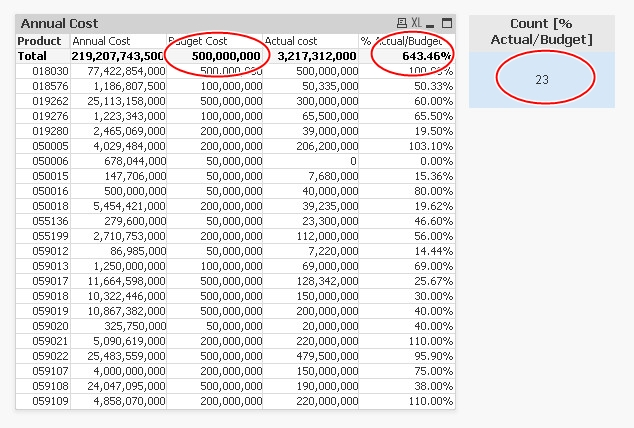# QlikView App Development

Discussion Board for collaboration related to QlikView App Development.

Announcements
Save the Date: QlikWorld Online, June 24-25, 2020. Free global virtual event for data integration and data analytic gurus. Register TodayNot applicable

## Total and Count IF of the Sum Result

Hi all,

I've been strugling over this TOTAL and COUNT IF problem. It should've been easy, but i just have no idea yet.

I've three pre-loaded field: Product, Annual Cost and Actual Cost.

Then in straigt-table i've to put Budget Cost, which have value range based on Annual Cost, like if the product have Annual Cost less than 900M, the Budget Cost value should 50M.

Budget Cost expression is as follows

=if((Sum([Annual Cost]))>1 and (Sum([Annual Cost]))<900000000, 50000000,

if((Sum([Annual Cost]))>900000000 and (Sum([Annual Cost]))<2400000000, 100000000,

if((Sum([Annual Cost]))>2400000000 and (Sum([Annual Cost]))<6000000000, 200000000,

if((Sum([Annual Cost]))>6000000000, 500000000

))))

And then i've to put [% Budget vs Actual], which the expression should be Actual/Budget. It's OK on the field, but not as i expect in TOTAL. Which lead incorrect percentage in TOTAL [% Budget vs Actual Cost]. See pic.

I expect the TOTAL would result the sum of the new-created Budget Cost.Then i have to put text field to show count ( if the [% Budget vs Actual] > 100% ). i've tried set analysis, and add variable (Actual/Budget), and write expression as follow:

=count({\$<v1={'>1'}>} Product)

Is it possible? Thanks in advance.

Tags (3)
3 Replies
Highlighted
Honored Contributor II

## Re: Total and Count IF of the Sum Result

In your % actual buget write expression and check on relative

hope it helps

HighlightedNot applicable

## Re: Total and Count IF of the Sum Result

my expression for %actual/budget is

=sum([Actual cost])/(if((Sum([Annual Cost]))>1 and (Sum([Annual Cost]))<900000000, 50000000,

if((Sum([Annual Cost]))>900000000 and (Sum([Annual Cost]))<2400000000, 100000000,

if((Sum([Annual Cost]))>2400000000 and (Sum([Annual Cost]))<6000000000, 200000000,

if((Sum([Annual Cost]))>6000000000, 500000000

)))))

in which per rows, give me correct answer. the incorrect TOTAL on %a/b is because the incorrect TOTAL of 'Budget Cost'. the 'Budget Cost' TOTAL is not summing the rows, but reflect the if's (the TOTAL is 500M because the 'actual' sum total is more than 6000M)

i tried 'relatif' option, but the answer is not yet my expectation.